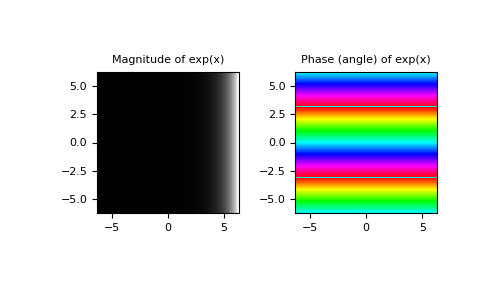# numpy.exp¶

numpy.exp(x, /, out=None, *, where=True, casting='same_kind', order='K', dtype=None, subok=True[, signature, extobj]) = <ufunc 'exp'>

Calculate the exponential of all elements in the input array.

Parameters
xarray_like

Input values.

outndarray, None, or tuple of ndarray and None, optional

A location into which the result is stored. If provided, it must have a shape that the inputs broadcast to. If not provided or None, a freshly-allocated array is returned. A tuple (possible only as a keyword argument) must have length equal to the number of outputs.

wherearray_like, optional

This condition is broadcast over the input. At locations where the condition is True, the out array will be set to the ufunc result. Elsewhere, the out array will retain its original value. Note that if an uninitialized out array is created via the default out=None, locations within it where the condition is False will remain uninitialized.

**kwargs

For other keyword-only arguments, see the ufunc docs.

Returns
outndarray or scalar

Output array, element-wise exponential of x. This is a scalar if x is a scalar.

expm1

Calculate exp(x) - 1 for all elements in the array.

exp2

Calculate 2**x for all elements in the array.

Notes

The irrational number e is also known as Euler’s number. It is approximately 2.718281, and is the base of the natural logarithm, ln (this means that, if $$x = \ln y = \log_e y$$, then $$e^x = y$$. For real input, exp(x) is always positive.

For complex arguments, x = a + ib, we can write $$e^x = e^a e^{ib}$$. The first term, $$e^a$$, is already known (it is the real argument, described above). The second term, $$e^{ib}$$, is $$\cos b + i \sin b$$, a function with magnitude 1 and a periodic phase.

References

1

Wikipedia, “Exponential function”, https://en.wikipedia.org/wiki/Exponential_function

2

M. Abramovitz and I. A. Stegun, “Handbook of Mathematical Functions with Formulas, Graphs, and Mathematical Tables,” Dover, 1964, p. 69, http://www.math.sfu.ca/~cbm/aands/page_69.htm

Examples

Plot the magnitude and phase of exp(x) in the complex plane:

>>> import matplotlib.pyplot as plt

>>> x = np.linspace(-2*np.pi, 2*np.pi, 100)
>>> xx = x + 1j * x[:, np.newaxis] # a + ib over complex plane
>>> out = np.exp(xx)

>>> plt.subplot(121)
>>> plt.imshow(np.abs(out),
...            extent=[-2*np.pi, 2*np.pi, -2*np.pi, 2*np.pi], cmap='gray')
>>> plt.title('Magnitude of exp(x)')

>>> plt.subplot(122)
>>> plt.imshow(np.angle(out),
...            extent=[-2*np.pi, 2*np.pi, -2*np.pi, 2*np.pi], cmap='hsv')
>>> plt.title('Phase (angle) of exp(x)')
>>> plt.show()